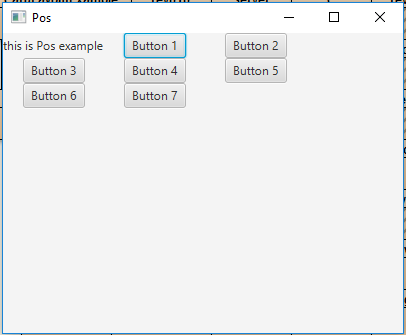# JavaFX | Pos Class

Pos class is a part of JavaFX. Pos class contains values which state the horizontal and vertical positioning or alignment. Pos class inherits Enum class.

Commonly Used Methods:

Method Explanation
getHpos() Returns the horizontal alignment.
getVpos() Returns the vertical alignment.
valueOf(String n) Returns the Pos object whose name is the string specified.
values() Returns an array which contains all the Pos values.

Below programs illustrate the use of Pos Class:

1. Java Program to create a tilepane and add a specified Pos value as its alignment: In this program we will create a TilePane named tile_pane. Add Label named label and some buttons to the tile_pane. Set the Alignment of the tile_pane using the setAlignment() function. Set the alignment of the tile_pane to the Pos value TOP_LEFT. Add the tile_pane to the scene and add the scene to the stage and call the show() function to display the final results.

 `// Java Program to create a tilepane and add ` `// a specified Pos value as its alignment ` `import` `javafx.application.Application; ` `import` `javafx.scene.Scene; ` `import` `javafx.scene.control.*; ` `import` `javafx.scene.layout.*; ` `import` `javafx.stage.Stage; ` `import` `javafx.geometry.*; ` `import` `javafx.scene.paint.*; ` `import` `javafx.scene.canvas.*; ` `import` `javafx.scene.text.*; ` `import` `javafx.scene.Group; ` `import` `javafx.scene.shape.*; ` ` `  `public` `class` `Pos_1 ``extends` `Application { ` ` `  `    ``// launch the application ` `    ``public` `void` `start(Stage stage) ` `    ``{ ` ` `  `        ``try` `{ ` ` `  `            ``// set title for the stage ` `            ``stage.setTitle(``"Pos"``); ` ` `  `            ``// create a label ` `            ``Label label = ``new` `Label(``"this is Pos example"``); ` ` `  `            ``// create a Tile pane ` `            ``TilePane tile_pane = ``new` `TilePane(label); ` ` `  `            ``// create and add buttons to tilepane ` `            ``for` `(``int` `i = ``1``; i <= ``7``; i++) { ` `                ``tile_pane.getChildren().add(``new` `Button(``"Button "` `+ i)); ` `            ``} ` ` `  `            ``// set Alignment of pane ` `            ``tile_pane.setAlignment(Pos.TOP_LEFT); ` ` `  `            ``// create a scene ` `            ``Scene scene = ``new` `Scene(tile_pane, ``400``, ``300``); ` ` `  `            ``// set the scene ` `            ``stage.setScene(scene); ` ` `  `            ``stage.show(); ` `        ``} ` ` `  `        ``catch` `(Exception e) { ` ` `  `            ``System.out.println(e.getMessage()); ` `        ``} ` `    ``} ` ` `  `    ``// Main Method ` `    ``public` `static` `void` `main(String args[]) ` `    ``{ ` ` `  `        ``// launch the application ` `        ``launch(args); ` `    ``} ` `} `

Output:2. Java Program to create a TilePane and a combobox that contains different values of Pos: In this program we will create a TilePane named tile_pane. Add Label named label and some buttons to the tile_pane. Set the Alignment of the tile_pane using the setAlignment() function. We will set the alignment of the tile_pane to the Pos value BASELINE_CENTER. Store all the names of Pos values in a String array. Now create a combobox which will contain the names of Pos values and also cranate a Action Event to handle the combobox events. The Event handler will set the Alignment of the tilepane to the chosen pos value. Now create a VBox and add the tilepane and the combo box to vbox. Finally, add the vbox to the scene and add the scene to the stage and call the show() function to display the final results.

 `// Java Program to create a TilePane and  ` `// a combobox that contains different  ` `// values of Pos ` `import` `javafx.application.Application; ` `import` `javafx.scene.Scene; ` `import` `javafx.scene.control.*; ` `import` `javafx.scene.layout.*; ` `import` `javafx.stage.Stage; ` `import` `javafx.geometry.*; ` `import` `javafx.scene.paint.*; ` `import` `javafx.scene.canvas.*; ` `import` `javafx.scene.text.*; ` `import` `javafx.scene.Group; ` `import` `javafx.scene.shape.*; ` `import` `javafx.event.ActionEvent; ` `import` `javafx.event.EventHandler; ` `import` `javafx.collections.*; ` `  `  `public` `class` `Pos_2 ``extends` `Application { ` `  `  `// launch the application ` `public` `void` `start(Stage stage) ` `{ ` ` `  `    ``try` `{ ` ` `  `        ``// set title for the stage ` `        ``stage.setTitle(``"Pos Class"``); ` ` `  `        ``// create a label ` `        ``Label label = ``new` `Label(``"This is Pos Class Example"``); ` ` `  `        ``// create a Tile pane ` `        ``TilePane tile_pane = ``new` `TilePane(label); ` ` `  `        ``// create and add buttons to tilepane ` `        ``for` `(``int` `i = ``1``; i <= ``7``; i++) { ` ` `  `            ``tile_pane.getChildren().add(``new` `Button(``"Button "` `+ i)); ` `        ``} ` ` `  `        ``// set Alignment of pane ` `        ``tile_pane.setAlignment(Pos.BASELINE_CENTER); ` ` `  `        ``// pos names ` `        ``String pos_name[] = {``"BASELINE_CENTER"``, ``"BASELINE_LEFT"``, ` `                             ``"BASELINE_RIGHT"``, ``"BOTTOM_CENTER"``,  ` `                             ``"BOTTOM_LEFT"``, ``"BOTTOM_RIGHT"``,  ` `                             ``"CENTER"``, ``"CENTER_LEFT"``, ``"CENTER_RIGHT"``, ` `                             ``"TOP_CENTER"``, ``"TOP_LEFT"``, ``"TOP_RIGHT"``}; ` ` `  `        ``// Create a combo box ` `        ``ComboBox combo_box =  ` `          ``new` `ComboBox(FXCollections.observableArrayList(pos_name)); ` ` `  `        ``// Create action event ` `        ``EventHandler event = ` `        ``new` `EventHandler() { ` ` `  `            ``public` `void` `handle(ActionEvent e) ` `            ``{ ` ` `  `                ``// set Alignement of the TilePane ` `                ``tile_pane.setAlignment(Pos.valueOf( ` `                     ``(String)combo_box.getValue())); ` `            ``} ` `        ``}; ` ` `  `        ``// Set on action ` `        ``combo_box.setOnAction(event); ` ` `  `        ``// create a VBox ` `        ``VBox vbox = ``new` `VBox(``30``, combo_box, tile_pane); ` ` `  `        ``// set Alignemnet ` `        ``vbox.setAlignment(Pos.CENTER); ` ` `  `        ``// create a scene ` `        ``Scene scene = ``new` `Scene(vbox, ``400``, ``300``); ` ` `  `        ``// set the scene ` `        ``stage.setScene(scene); ` ` `  `        ``stage.show(); ` `    ``} ` ` `  `    ``catch` `(Exception e) { ` ` `  `        ``System.out.println(e.getMessage()); ` `    ``} ` `} ` ` `  `// Main Method ` `public` `static` `void` `main(String args[]) ` `{ ` ` `  `    ``// launch the application ` `    ``launch(args); ` `} ` `} `

Output:

Note: The above programs might not run in an online IDE please use an offline compiler.My Personal Notes arrow_drop_upCheck out this Author's contributed articles.

If you like GeeksforGeeks and would like to contribute, you can also write an article using contribute.geeksforgeeks.org or mail your article to contribute@geeksforgeeks.org. See your article appearing on the GeeksforGeeks main page and help other Geeks.

Please Improve this article if you find anything incorrect by clicking on the "Improve Article" button below.

Article Tags :
Practice Tags :

Be the First to upvote.

Please write to us at contribute@geeksforgeeks.org to report any issue with the above content.